# Six Phase Wound Rotor Synchronous Machine (Double Stator)

Description of the Six Phase Wound Rotor Synchronous Machine (Double Stator) component in Schematic Editor.

Table 1. Six Phase Wound Rotor Synchronous Machine (Double Stator) component in Typhoon HIL Schematic Editor
component component dialog window component parameters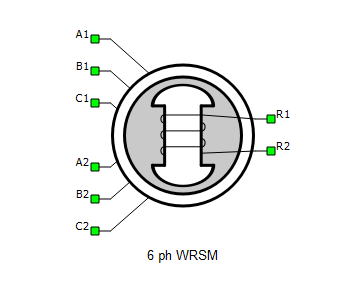• Property tabs:

A1, B1, and C1 are the stator winding 1 terminals. A2, B2, and C2 are the stator winding 2 terminals. Stator windings use the current source interface. R1 and R2 are the field winding terminals. Both damper windings on the rotor are short-circuited and without output terminals. The rotor winding uses the single phase variant of the current source interface.

## Electrical sub-system model

The electrical part of the machine is represented by the following system of equations, modeled in the rotating dq reference frame. The dq reference frame is attached to the rotor, and the direct axis is aligned with the field winding axis.

$\left[\begin{array}{c}{v}_{ds1}\\ {v}_{qs1}\\ {v}_{ds2}\\ {v}_{qs2}\\ {v}_{fd}\\ {v}_{kd}=0\\ {v}_{kq}=0\end{array}\right]=\left[\begin{array}{ccccccc}{R}_{s1}& 0& 0& 0& 0& 0& 0\\ 0& {R}_{s1}& 0& 0& 0& 0& 0\\ 0& 0& {R}_{s2}& 0& 0& 0& 0\\ 0& 0& 0& {R}_{s2}& 0& 0& 0\\ 0& 0& 0& 0& {R}_{fd}& 0& 0\\ 0& 0& 0& 0& 0& {R}_{kd}& 0\\ 0& 0& 0& 0& 0& 0& {R}_{kq}\end{array}\right]\left[\begin{array}{c}{i}_{ds1}\\ {i}_{qs1}\\ {i}_{ds2}\\ {i}_{qs2}\\ {i}_{fd}\\ {i}_{kd}\\ {i}_{kq}\end{array}\right]+\frac{d}{dt}\left[\begin{array}{c}{\psi }_{ds1}\\ {\psi }_{qs1}\\ {\psi }_{ds2}\\ {\psi }_{qs2}\\ {\psi }_{fd}\\ {\psi }_{kd}\\ {\psi }_{kq}\end{array}\right]+\left[\begin{array}{c}{-\omega }_{r}{\psi }_{qs1}\\ {\omega }_{r}{\psi }_{ds1}\\ {-\omega }_{r}{\psi }_{qs2}\\ {\omega }_{r}{\psi }_{ds2}\\ 0\\ 0\\ 0\end{array}\right]$

$\left[\begin{array}{c}{\psi }_{ds1}\\ {\psi }_{qs1}\\ {\psi }_{ds2}\\ {\psi }_{qs2}\\ {\psi }_{fd}\\ {\psi }_{kd}\\ {\psi }_{kq}\end{array}\right]=\left[\begin{array}{ccccccc}{L}_{ls1}+{L}_{ls12}+{L}_{md}& 0& {L}_{ls12}+{L}_{md}& 0& {L}_{md}& {L}_{md}& 0\\ 0& {L}_{ls1}+{L}_{ls12}+{L}_{mq}& 0& {L}_{ls12}+{L}_{mq}& 0& 0& {L}_{mq}\\ {L}_{ls12}+{L}_{md}& 0& {L}_{ls2}+{L}_{ls12}+{L}_{md}& 0& {L}_{md}& {L}_{md}& 0\\ 0& {L}_{ls12}+{L}_{mq}& 0& {L}_{ls2}+{L}_{ls12}+{L}_{mq}& 0& 0& {L}_{mq}\\ {L}_{md}& 0& {L}_{md}& 0& {L}_{lfd}+{L}_{md}& {L}_{md}& 0\\ {L}_{md}& 0& {L}_{md}& 0& {L}_{md}& {L}_{lkd}+{L}_{md}& 0\\ 0& {L}_{mq}& 0& {L}_{mq}& 0& 0& {L}_{lkq}+{L}_{mq}\end{array}\right]\left[\begin{array}{c}{i}_{ds1}\\ {i}_{qs1}\\ {i}_{ds2}\\ {i}_{qs2}\\ {i}_{fd}\\ {i}_{kd}\\ {i}_{kq}\end{array}\right]$

${T}_{e}=\frac{3}{2}p\left({\psi }_{ds1}{i}_{qs1}-{\psi }_{qs1}{i}_{ds1}+{\psi }_{ds2}{i}_{qs2}-{\psi }_{qs2}{i}_{ds1}\right)$

All rotor variables and parameters are referred to the stator with an appropriate turns ratio. To illustrate this, if you apply voltage vfdr at the field winding terminals, it is transformed to the stator value vfd with the following equation:

${v}_{fd}=\frac{{N}_{s}}{{N}_{fd}}{v}_{fd}^{r}$

Similarly, the calculated rotor currents (field winding current ifd and damper winding currents ikd and ikq) are referred back to the real rotor windings values ifdr, ikdr, and ikqr, through the following equations:

Table 2. Electrical subsystem model variables
symbol description
ψds1 Direct axis component of the stator winding 1 flux [Wb]
ψqs1 Quadrature axis component of the stator winding 1 flux [Wb]
ψds2 Direct axis component of the stator winding 2 flux [Wb]
ψqs2 Quadrature axis component of the stator winding 2 flux [Wb]
ψkd Direct axis component of the damper winding flux, referred to the stator [Wb]
ψkq Quadrature axis component of the damper winding flux, referred to the stator [Wb]
ψfd Field winding flux, referred to the stator [Wb]
ids1 Direct axis component of the stator winding 1 current [A]
iqs1 Quadrature axis component of the stator winding 1 current [A]
ids2 Direct axis component of the stator winding 2 current [A]
iqs2 Quadrature axis component of the stator winding 2 current [A]
ikd Direct axis component of the damper winding current, referred to the stator [A]
ikdr Direct axis component of the damper winding current [A]
ikq Quadrature axis component of the damper winding current, referred to the stator [A]
ikqr Quadrature axis component of the damper winding current [A]
ifd Field winding current, referred to the stator [A]
ifdr Field winding current [A]
vds1 Direct axis component of the stator winding 1 voltage [V]
vqs1 Quadrature axis component of the stator winding 1 voltage [V]
vds2 Direct axis component of the stator winding 2 voltage [V]
vqs2 Quadrature axis component of the stator winding 2 voltage [V]
vfd Field winding voltage, referred to the stator [V]
vfdr Field winding voltage [V]
vkd Direct axis component of the damper winding voltage, referred to the stator [V]
vkq Quadrature axis component of the damper winding voltage, referred to the stator [V]
Rs1 Stator winding 1 phase resistance [Ω]
Rs2 Stator winding 2 phase resistance [Ω]
Rkd Direct axis damper winding resistance, referred to the stator [Ω]
Rkq Quadrature axis damper winding resistance, referred to the stator [Ω]
Rfd Field winding resistance, referred to the stator [Ω]
Lls1 Stator winding 1 phase leakage inductance [H]
Lls2 Stator winding 2 phase leakage inductance [H]
Lls12 Common leakage inductance of stator windings 1 and 2 [H]
Lmd Direct axis magnetizing (mutual, main) inductance [H]
Lmq Quadrature axis magnetizing (mutual, main) inductance [H]
Llkd Direct axis damper winding leakage inductance, referred to the stator [H]
Llkq Quadrature axis damper winding leakage inductance, referred to the stator [H]
Llfd Direct axis field winding leakage inductance, referred to the stator [H]
ωr Rotor electrical speed [rad/s] ( $=p{\omega }_{m}$ )
p Machine number of pole pairs
Te Machine developed electromagnetic torque [Nm]

## Mechanical sub-system model

Motion equation:

Table 3. Mechanical sub-system model variables
symbol description
Jm Combined rotor and load moment of inertia [kgm2]
Te Machine developed electromagnetic torque [Nm]
Tl Shaft mechanical load torque [Nm]
b Machine viscous friction coefficient [Nms]
Note: Motion equation is the same for all of the rotating machine models.

## Electrical

Table 4. Electrical parameters
symbol description
Rs1 Stator winding 1 phase resistance [Ω]
Rs2 Stator winding 2 phase resistance [Ω]
Lls1 Stator winding 1 phase leakage inductance [H]
Lls2 Stator winding 2 phase leakage inductance [H]
Lls12 Common leakage inductance of stator windings 1 and 2 [H]
Lmd Direct axis magnetizing (mutual, main) inductance [H]
Lmq Quadrature axis magnetizing (mutual, main) inductance [H]
Rf Field winding resistance, referred to the stator [Ω]
Llfd Direct axis field winding leakage inductance, referred to the stator [H]
Rkd Direct axis damper winding resistance, referred to the stator [Ω]
Rkq Quadrature axis damper winding resistance, referred to the stator [Ω]
Llkd Direct axis damper winding leakage inductance, referred to the stator [H]
Llkq Quadrature axis damper winding leakage inductance, referred to the stator [H]
Ns/Nfd Turns ratio between the stator phase winding and the field winding used for transforming field winding variables to the stator-side
Ns/Nkd Turns ratio between the stator phase winding and the direct axis damper winding used for transforming damper winding variables to the stator-side
Ns/Nkq Turns ratio between the stator phase winding and the quadrature axis damper winding used for transforming damper winding variables to the stator-side

## Mechanical

Table 5. Mechanical parameters
symbol description
pms Machine number of pole pairs
Star/delta w1 Stator winding 1 connection (star or delta)
Star/delta w2 Stator winding 2 connection (star or delta)
Jm Combined rotor and load moment of inertia [kgm2]
Friction coefficient Machine viscous friction coefficient [Nms]
Unconstrained mechanical angle Limiting mechanical angle between 0 and 2π

symbol description
Load source Load can be set from SCADA/external or from model (in model case, one signal processing input will appear)
Load ai offset Assigned offset value to the input signal representing external torque command
Load ai gain Assigned gain value to the input signal representing external torque command

External load enables you to use an analog input signal from a HIL analog channel with the load_ai_pin address as an external torque/speed load, and to assign offset (V) and gain (Nm/V) to the input signal, according to the formula:

${T}_{l}=load_ai_gain·\left(AI\left(load_ai_pin\right)+load_ai_offset\right)$

Note: An analog input pin may be overwritten if another component uses the same analog input pin. If another property (from the same or a different component) uses the same analog input pin, the input signal value will be applied to only one of those properties. For instance, if both the load and the resolver carrier signal use the same analog input pin, the signal value will only be applied only to one of these.

## Feedback

Table 7. Feedback parameters
symbol description
Encoder ppr Incremental encoder number of pulses per revolution
Resolver pole pairs Resolver number of pole pairs
Resolver carrier source Resolver carrier signal source selection (internal or external)
External resolver carrier source type External resolver carrier signal source type selection (single ended or differential); available only if the Resolver carrier source property is set to external
Resolver carrier frequency Resolver carrier signal frequency (internal carrier) [Hz]
Resolver ai pin 1 Resolver carrier input channel 1 address (external carrier)
Resolver ai pin 2 Resolver carrier input channel 2 address (external carrier); available only if the External resolver carrier source type property is set to differential
Resolver ai offset Resolver carrier input channel offset (external carrier)
Resolver ai gain Resolver carrier input channel gain (external carrier)
Absolute encoder protocol Standardized protocol providing the absolute machine encoder position

If an external resolver carrier source is selected, the source signal type can be set as either single ended or differential. The single ended external resolver carrier source type enables use of an analog input signal from the HIL analog channel with the res_ai_pin_1 address as the external carrier source. Additionally, offset (V) and gain (V/V) values can be assigned to the input signal, according to the formula:

$res_carr_src=res_ai_gain·\left(AI\left(res_ai_pin_1\right)+res_ai_offset\right)$

The differential external resolver carrier source type enables use of two analog input signals from the HIL analog channels with the res_ai_pin_1 and the res_ai_pin_2 addresses. Analog signals from these HIL analog inputs are subtracted, and the resulting signal is used as the external differential carrier source. Additionally, offset (V) and gain (V/V) values can be assigned to the input signal (similarly to the single ended case), according to the formula:

$res_carr_src=res_ai_gain·\left(\left(AI\left(res_ai_pin_1\right)-AI\left(res_ai_pin_2\right)\right)+res_ai_offset\right)$
Note: Differential external resolver signal support is available from the 2023.1 software version. Only the single ended external resolver option was available in previous software versions.
Note: In order to get a resolver signal with an amplitude of 1 when using an external carrier signal, the offset and the gain should be chosen in such a way that the resolver carrier signal has an amplitude of 1 after the adjustment. As shown in Figure 1, the sinusoidal signal used to generate external resolver carrier source is fed to HIL analog input 1. The analog input signal is scaled in order to get the resolver signals with an amplitude of 1.
Note: An analog input pin may be overwritten if another component uses the same analog input pin. If another property (from the same or a different component) uses the same analog input pin, the input signal value will be applied to only one of those properties. E.g. if both the load and the resolver carrier signal use the same analog input pin, the signal value will be applied only to one of these.

The following expression must hold in order to properly generate the encoder signals:

$4·enc_ppr·{f}_{m}{·T}_{s}\le 1$
Table 8. Variables in the encoder limitation expression
symbol description
enc_ppr Encoder number of pulses per revolution
fm Rotor mechanical frequency [Hz]
Ts Simulation time step [s]
Note: While the machine speed is positive, the encoder channel B signal leads the encoder channel A signal.
Note: Absolute encoder protocol is not supported on HIL402 (configurations: 1, 2, 3, and 4).

symbol description
Theta_ab Position of the stationary αβ reference frame, in respect to the stator phase a axis [rad]

The machine model output variables (currents, voltages and fluxes) can be observed from a stationary reference frame. There are two widely used approaches in electrical machine modeling: in the first, the alpha axis of the stationary reference frame lags by 90 degrees in regard to the stator phase a axis (used by default, and indicated in a) Figure 5. In the second one, the alpha axis is aligned with the stator phase a axis (indicated in b) Figure 5. The user can select between these two situations.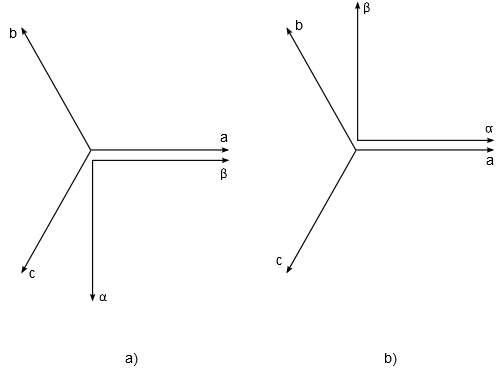Figure 3. Selection of a stationary reference frame position: a) Theta_ab= -pi/2; b) Theta_ab= 0

It is important to know the value of Theta_ab when the rotor position feedback is necessary. As an example, if a model uses the mechanical angle as a feedback signal and feeds it to one of the abc to dq, alpha beta to dq, dq to abc, or dq to alpha beta transformation blocks, the same transformation angle offset value should be used in both components to ensure the expected simulation results.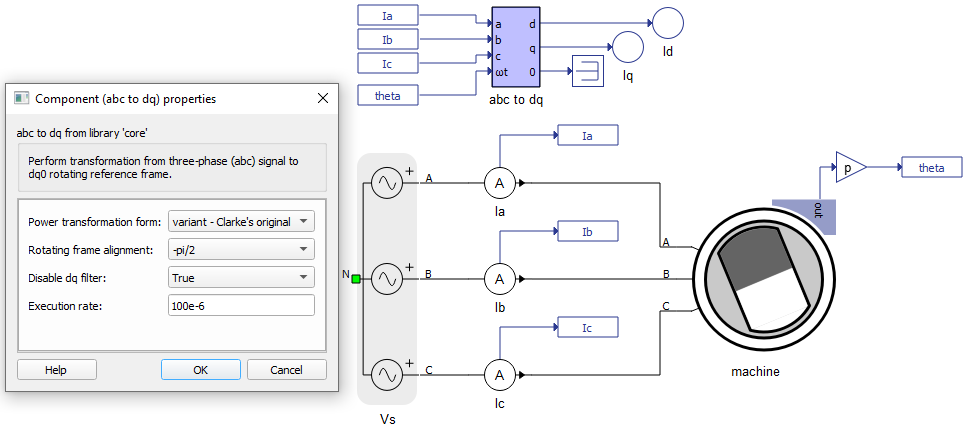Figure 4. Example of model containing abc to dq transformation component and chosen stationary reference frame position Theta_ab= -pi/2
Note: This property is available only on certain machine components.

## Snubber

All machines with current source based circuit interfaces have the Snubber tab in the properties window where the value of snubber resistance can be set. Snubbers are necessary in the cases when an inverter or a contactor is directly connected to the machine terminals. This value can be set to infinite (inf), but it is not recommended when a machine is directly connected to the inverter since there will be a current source directly connected to an open switch. In this case, one of each switch pairs S1 and S2, S3 and S4, and S5 and S6 will be forced closed by the circuit solver in order to avoid the topological conflicts. On the other hand, with finite snubber values, there's always a path for the currents Ia and Ib, so all inverter switches can be open in this case. Circuit representations of this circuit without and with snubber resistors are shown in Figure 3 and Figure 4 respectively. Snubbers are connected across the current sources.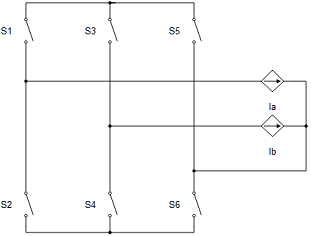Figure 5. Circuit representation of machine and inverter when all the switches are open without resistors (not recommended)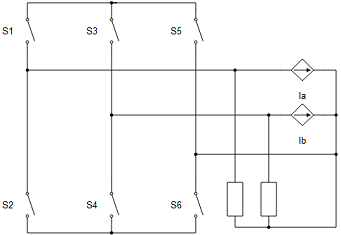Figure 6. Circuit representation of machine and inverter when all the switches are open with resistors (recommended)
Note: Snubbers exist only in the machine components that have the current source based circuit interface.
Note: Snubbers are dynamic, which means that the snubber is dynamically added to circuit modes where topological conflicts are detected.
Table 10. Snubber parameters
symbol description
Rsnb rotor Rotor snubber resistance value [Ω]
Rsnb stator w1 Stator winding 1 snubber resistance value [Ω]
Rsnb stator w2 Stator winding 2 snubber resistance value [Ω]

## Output

This block tab enables a single, vectorized signal output from the machine. The output vector contains selected machine mechanical and/or electrical variables in the same order as listed in this tab.

Note: All machine components have the Execution rate, Electrical torque, Mechanical speed, and Mechanical angle, but the rest of the signals differ from component to component.
Table 11. Output parameters
symbol description
Execution rate Signal processing output execution rate [s]
Electrical torque Machine electrical torque [Nm]
Mechanical speed Machine mechanical angular speed [rad/s]
Mechanical angle Machine mechanical angle [rad]
Stator 1 d-axis current Direct axis component of the stator 1 current [A]
Stator 1 q-axis current Quadrature axis component of the stator 1 current [A]
Stator 1 d-axis flux Direct axis component of the stator 1 flux [Wb]
Stator 1 q-axis flux Quadrature axis component of the stator 1 flux [Wb]
Stator 2 d-axis current Direct axis component of the stator 2 current [A]
Stator 2 q-axis current Quadrature axis component of the stator 2 current [A]
Stator 2 d-axis flux Direct axis component of the stator 2 flux [Wb]
Stator 2 q-axis flux Quadrature axis component of the stator 2 flux [Wb]